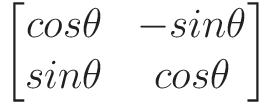Select Page

# CBSE Maths 12 Science MCQ Application of Derivatives Solutions in English

CBSE Maths 12 Science MCQ Application of Derivatives Solutions in English to enable students to get Solutions in a narrative video format for the specific question.

Expert Teacher provides CBSE Maths 12 Science MCQ Application of Derivatives Solutions through Video Solutions in English language. This video solution will be useful for students to understand how to write an answer in exam in order to score more marks. This teacher uses a narrative style for a question from Application of Derivatives not only to explain the proper method of answering question, but deriving right answer too.

Please find the question below and view the Solution in a narrative video format.

Question:

Solution Video in English:

You can select video Solutions from other languages also. Please check Solutions in ( Hindi )

## Similar Questions from CBSE, 12th Science, Maths, Application of Derivatives

Question 1 : Find the maximum value of inthe interval [1,3]. find the maximum value of the same function in [-3,-1]. (View Answer Video)

Question 2 : Find approximate value of. (View Answer Video)

Question 3 : A balloon, which always remains spherical has a variable radius. Find the rate at which its volume is increasing with the radius when the later is 10 cm.  (View Answer Video)

Question 4 : For all values of x, the minimum value ofis : (View Answer Video)

Question 5 : If, then the approximate value of f (3.02) is  (View Answer Video)

### Three Dimensional Geometry

Question 1 : Find the angle between the lines whose direction ratios are a, b, c and b - c, c - a, a - b. (View Answer Video)

Question 2 : Write the vector equation of a line passing through the point (1, -1, 2) and parallel to the line whose equations are,(View Answer Video)

Question 3 : Find the distance of the point (-1, -5, -10) from the point of intersection of the lineand the plane(View Answer Video)

Question 5 : If a line has direction ratios 2, -1, -2, then what are its direction cosines? (View Answer Video)

### Determinant

Question 1 : Evaluate  the determinants in :.(View Answer Video)

Question 2 : A system of linear equations AX = B is said to be inconsistent, if the system of equations has, (View Answer Video)

Question 3 :  Using the properties of determinants, evaluate. (View Answer Video)

Question 4 : If, then x is equal to, (View Answer Video)

Question 5 : Evaluate. (View Answer Video)

### Relations and Functions

Question 1 : Ifis a relation on N, write the range of R. (View Answer Video)

Question 2 : Number of binary operations on the set { a, b} are: (View Answer Video)

Question 3 : The law a + b = b + a is called ______. (View Answer Video)

Question 4 : Number of binary operations on the set {a, b} are : (View Answer Video)

Question 5 : Let R be the relation on the set N given by R ={(a, b): a = b - 2, b > 6}. Choose the correct answer. (View Answer Video)